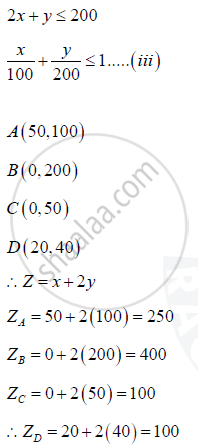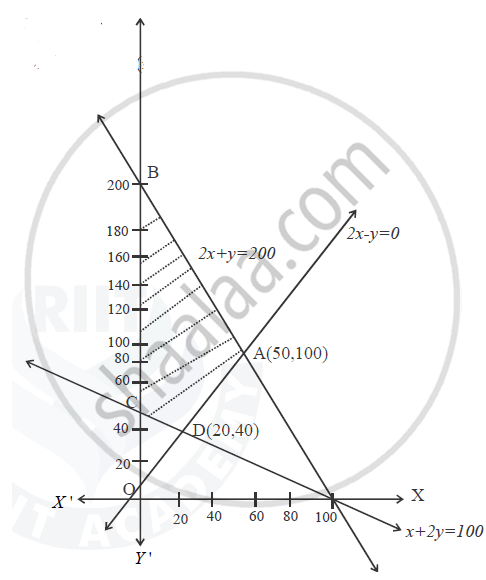Share

# Maximise Z = X + 2y Subject to the Constraints X + 2y >= 10 2x - Y <= 0 and 2x + Y <= 20 Solve the Above Lpp Graphically - CBSE (Commerce) Class 12 - Mathematics

ConceptGraphical Method of Solving Linear Programming Problems

#### Question

Maximise Z = x + 2y subject to the constraints

x + 2y >= 100

2x - y <= 0

2x + y <= 200

Solve the above LPP graphically

#### Solution

x + 2y ≥ 100

x/100 + y/50 >= 1 ....(i)

2x - y <= 0

 x 10 20 30 y 20 40 60 (x,y) (10,20) (20,40) (30,60)∴ Z is maximum at 0, 200 & max Z = 400Is there an error in this question or solution?

#### Video TutorialsVIEW ALL 

Solution Maximise Z = X + 2y Subject to the Constraints X + 2y >= 10 2x - Y <= 0 and 2x + Y <= 20 Solve the Above Lpp Graphically Concept: Graphical Method of Solving Linear Programming Problems.
S